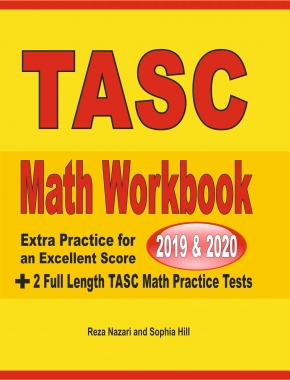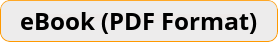\$15.99

# TASC Math Workbook 2019 & 2020 Extra Practice for an Excellent Score + 2 Full Length TASC Math Practice TestsLooking for a comprehensive TASC Math workbook to help you succeed on the TASC Math test? Need a great TASC Math workbook for teaching, tutoring and preparing students for the TASC Math test? If so, then this great math workbook is just for you.Teacher's Choice
100% Guaranteed
Secure Checkout

### A Comprehensive Math Workbook for the TASC Test!

The surest way to succeed on the TASC Math Test is with intensive practice in every math topic tested–and that’s what you will get in TASC Math Workbook 2019 & 2020.

This perfect workbook with more than 2,500 sample questions and 2 complete TASC Mathematics practice tests is all you need to measure your exam-readiness, find your weak areas, and learn from your mistakes.

Separate math chapters in this workbook offer a complete review of the TASC Math test, including:

• Arithmetic and Number Operations
• Algebra and Functions,
• Geometry and Measurement
• Data analysis, Statistics, & Probability
• … and also includes 2 realistic TASC Math practice tests!

Written by Effortless Math Education TASC Math experts and top TASC instructors, this great math workbook left no stone unturned. The content of this book is 100% aligned with the 2019 and 2020 TASC test guidelines.

ACE the TASC Math Test with a COMPREHENSIVE Math Workbook!